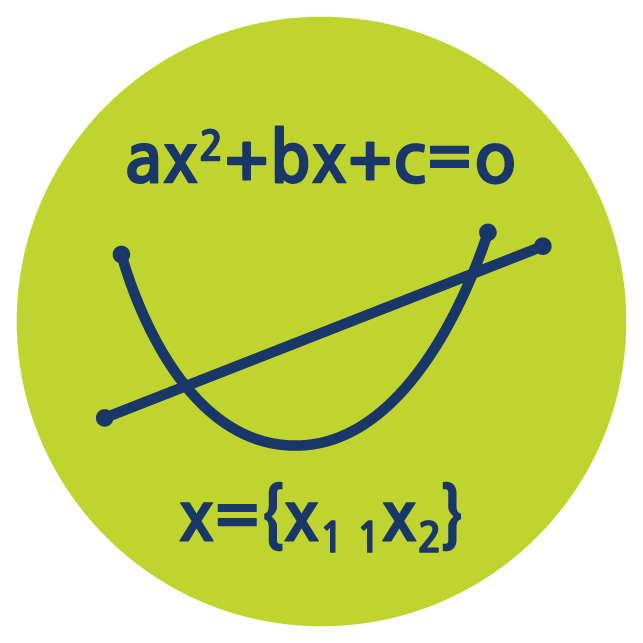Select Page# Solving Equations

The Solving Equations online course helps you develop core algebra techniques and then solve various types of equation. Equations are how solutions to Math problems are presented so this is a key skill for all subsequent Math courses. Master solving equations now and make all future Math courses significantly easier. View our classroom with this PREVIEW or learn more below and start your FREE one week Solving Equations TRIAL.

### Who is the Solving Equations Online Course For?

Solving equations is unquestionably one of the most important Math skills, so the sooner students master these techniques the better. The most basic equations taught in this course are accessible for students with little or no algebra experience. However, the more challenging types will require students to have taken Algebra 1, or a similar course. View the CLASSROOM.

### What is Taught in the Solving Equations Online Course?

The solving equations online course helps students master equations even if they are completely new to the subject. Otherwise, the course can also be used by experienced students to review and practice key techniques whenever required using our on-demand videos and practice worksheets. View the full Solving Equations online course SYLLABUS.

### What Will I Learn in This Course?

Students will firstly learn the algebra techniques required to solve a range of equation types and then solve the equations themselves. These skills are foundational for all subsequent Math courses in Pre-Calculus & Calculus. Students learn key techniques and common question types from our step by step instructional videos and then practice using our carefully designed worksheets.

### How Does Solving Equations Compare to Algebra 1?

Certainly, there is some cross-over between Algebra 1 and Solving Equations. However, whereas Algebra 1 covers a range of algebra techniques, Solving Equations focuses only on the algebra require for solving equations. Algebra 1 is a particularly great pre-requisite for Solving Equations. Learn more about ALGEBRA 1.

### What’s Next?

Although Solving Equations could be considered a stand-alone course, it teaches techniques which are a good foundation for Algebra 2 and mastering these will make all subsequent Math courses easier.

## Algebra to Pre-Calculus Course Bundle

Access all 6 courses in the bundle Algebra to Pre-Calculus to easily review older topics or jump ahead when ready.